Linear Algebra - 1

# Linear Algebra - 1

Test Description

## 10 Questions MCQ Test Question Bank for GATE Computer Science Engineering | Linear Algebra - 1

Linear Algebra - 1 for Computer Science Engineering (CSE) 2022 is part of Question Bank for GATE Computer Science Engineering preparation. The Linear Algebra - 1 questions and answers have been prepared according to the Computer Science Engineering (CSE) exam syllabus.The Linear Algebra - 1 MCQs are made for Computer Science Engineering (CSE) 2022 Exam. Find important definitions, questions, notes, meanings, examples, exercises, MCQs and online tests for Linear Algebra - 1 below.
Solutions of Linear Algebra - 1 questions in English are available as part of our Question Bank for GATE Computer Science Engineering for Computer Science Engineering (CSE) & Linear Algebra - 1 solutions in Hindi for Question Bank for GATE Computer Science Engineering course. Download more important topics, notes, lectures and mock test series for Computer Science Engineering (CSE) Exam by signing up for free. Attempt Linear Algebra - 1 | 10 questions in 30 minutes | Mock test for Computer Science Engineering (CSE) preparation | Free important questions MCQ to study Question Bank for GATE Computer Science Engineering for Computer Science Engineering (CSE) Exam | Download free PDF with solutions
 1 Crore+ students have signed up on EduRev. Have you?
Linear Algebra - 1 - Question 1

### A square matrix is singular whenever:

Detailed Solution for Linear Algebra - 1 - Question 1

If the rows (or columns) of a square matrix are linearly dependent, then the determinant of matrix becomes zero.
Therefore, whenever the rows are linearly dependent, the matrix is singular.

Linear Algebra - 1 - Question 2

### If A and B are real symmetric matrices of size n x n. Then, which one of the following is true?

Detailed Solution for Linear Algebra - 1 - Question 2

The matrix M is s aid to be sym m etric iff MT= M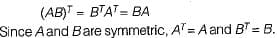Linear Algebra - 1 - Question 3

### Let a = (aij) be an n-rowed square matrix and I12 be the matrix obtained by interchanging the first and second rows of the n-rowed Identity matrix. Then AI12 is such that its first

Detailed Solution for Linear Algebra - 1 - Question 3

Let,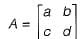I12 is the matrix obtained by inter-changing the first and second row of the identity Matrix I.
So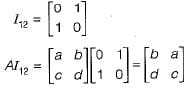AI12 is the matrix having first column same as the second column of A.

Linear Algebra - 1 - Question 4

The rank of the matrix given below is: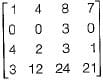Detailed Solution for Linear Algebra - 1 - Question 4

The given matrix is: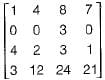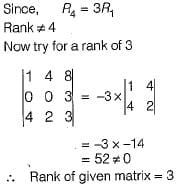Linear Algebra - 1 - Question 5

Consider the following determinant: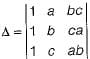Which of the following is a factor of Δ?

Detailed Solution for Linear Algebra - 1 - Question 5

The determinant of a matrix can’t be affected by elementary row operation
So,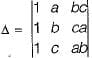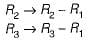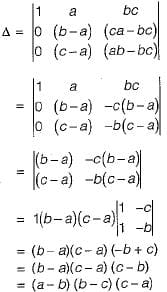So (a - b) is a factor of Δ.

Linear Algebra - 1 - Question 6

The rank of the matrix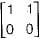is

Detailed Solution for Linear Algebra - 1 - Question 6

The given matrix isAbove matrix has only 1 independent row, so the given matrix has rank 1.

Linear Algebra - 1 - Question 7

The following system of equations: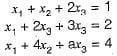Has a unique solution. The only possible value(s) for a is/are

Detailed Solution for Linear Algebra - 1 - Question 7

The augmented matrix for above system is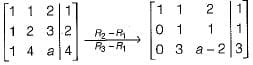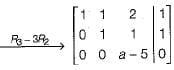Now as long as a - 5 ≠ 0,
rank (A) = rank (A | B) = 3
∴ a can take any real value except 5.

Linear Algebra - 1 - Question 8

If M is a square matrix with a zero determinant, which of the following assertion(S) is (are) correct?
S1: Each row of M can be represented as a linear combination of the other rows.
S2: Each column of M can be represented as a linear combination of the other columns.
S3: MX = O has a nontrivial solution.
S4: M has an inverse.

Detailed Solution for Linear Algebra - 1 - Question 8

S1 and S2:
Since M has zero determinant, its rank is not full i.e. if M is of size 3 x 3 , then its rank is not 3. So there is a linear combination of rows which evaluates to 0 i.e. k1R1 + k2R+...+knRn = 0 and there is a linear combination of columns which evaluates to 0 i.e.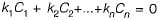Now any row Ri can be written as linear combination of other rows as: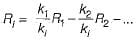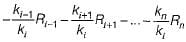Similar is the case for columns.
So S1 and S2 are true.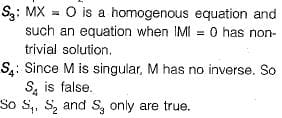Linear Algebra - 1 - Question 9

Consider the matrix as given below: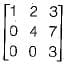Which one of the following options provides the CORRECT values of the eigenvalues of the matrix?

Detailed Solution for Linear Algebra - 1 - Question 9

Since the given matrix is upper triangular, its eigen values are the diagonal elements themselves, which are 1, 4 and 3.

Linear Algebra - 1 - Question 10

Consider the following 2 x 2 matrix A where two elements are unknown and are marked by ‘a’ and ‘b'. The eigenvalues of this matrix are -1 and 7. What are the values of ‘a’ and 'b'?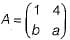Detailed Solution for Linear Algebra - 1 - Question 10

Trace = Sum of eigen values
1 + a = 6
⇒ a = 5
Determinant = Product of eigen values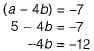⇒ b = 3
∴ a = 5, b = 3

## Question Bank for GATE Computer Science Engineering

61 videos|7 docs|102 tests
 Use Code STAYHOME200 and get INR 200 additional OFF Use Coupon Code
Information about Linear Algebra - 1 Page
In this test you can find the Exam questions for Linear Algebra - 1 solved & explained in the simplest way possible. Besides giving Questions and answers for Linear Algebra - 1, EduRev gives you an ample number of Online tests for practice

## Question Bank for GATE Computer Science Engineering

61 videos|7 docs|102 tests